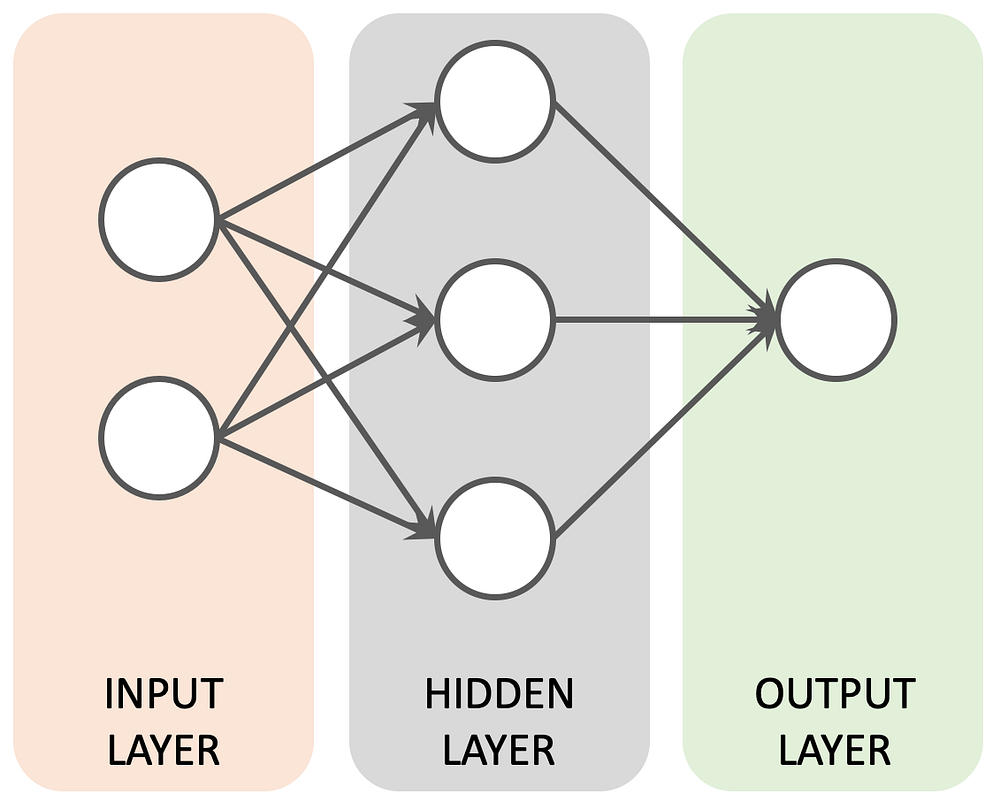# Creating a Artificial Neural Network from scratch using C#

Original article can be found here (source): Deep Learning on Medium# Let’s build Artificial Neural Network in C#! How hard can it be?

Developing models using C# is easy and fun, but real understanding can be achieved only via reading and implementing the algorithms on your own, build a Neural Network (shallow one) from scratch, using only pure C#. The real challenge is to implement the core algorithm that is used to train (Deep) Neural Networks — Back propagation. Shall we start?

# Setup

Let’s begin by preparing our environment and seeding the random number generator properly using below code.

# Background

## Input with XOR Gates

We will try to create an Artificial Neural Network (ANN) that can properly predict values from the XOR function. Here is its truth table for XOR Gates:

# Feedforward

This is the simplest form of ANN (artificial neural network); data travels only in one direction (input to output). This is the example we just looked at. When you actually use it, it’s fast; when you’re training it, it takes a while. Almost all vision and speech recognition applications use some form of this type of neural network.

Feedforward neural networks are artificial neural networks where the connections between units do not form a cycle. Feedforward neural networks were the first type of artificial neural network invented and are simpler than their counterpart, recurrent neural networks. They are called feedforward because information only travels forward in the network (no loops), first through the input nodes, then through the hidden nodes (if present), and finally through the output nodes.

Process of Feedforward neural network summing of all inputs with weights applying an activation function like Sigmoid Function and finally receiving the outputs.